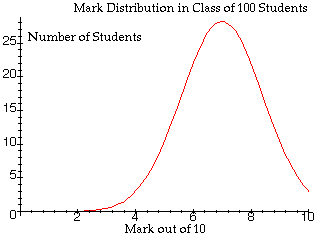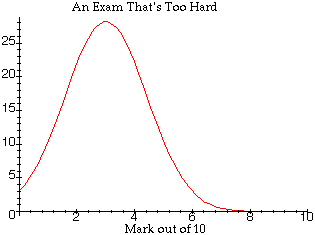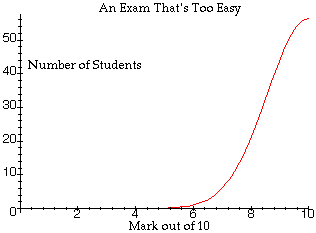# CLAW FAQ - What is a Bell Curve?

 A "bell curve" is the common name of a Gaussian Probability Distribution, because is is shaped like a bell. In a large class with a fair exam, the marks will roughly follow a Gaussian Probability Distribution. An example is shown to the right, for a class of 100 students and an exam marked out of 10. The average mark is 7 out of 10.When a professor crafts an exam, the objective is to have a fair distribution of marks which is roughly Gaussian. However, it doesn't always work out that way. If the exam is too hard, the marks distribution might look more like this example shown to the right. The average mark is only 3 out of 10.Sometimes a professor makes an exam too easy. In which case the marks distribution might look like this example shown to the right. The distribution is no longer even Gaussian. These problems are compensated for, by what is commonly referred to as "curving the marks." For more information about how this is done, see the CLAW FAQ - How are the marks curved?SFU / Math & Stats / ~hebron / claw / faq / bellcurve.html

Revised 21 July 1999 by John Hebron.